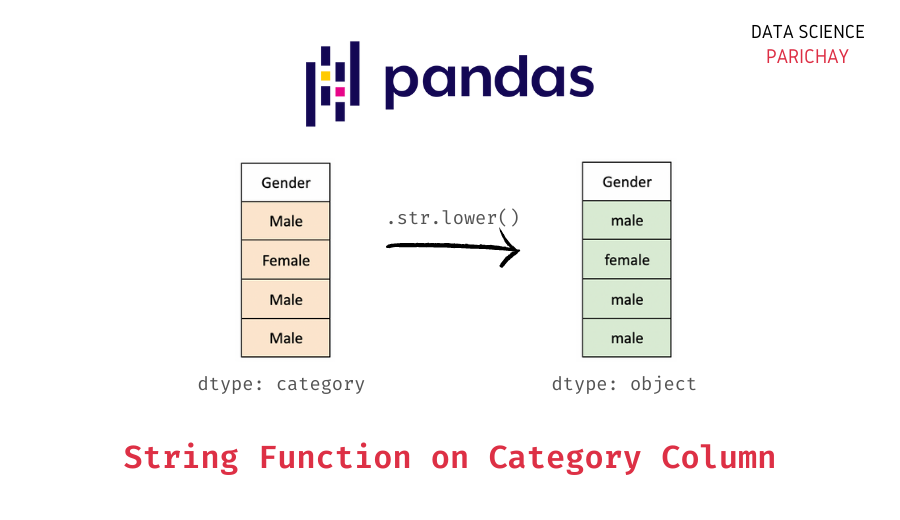# Pandas – Apply String Functions to Category Column

In this tutorial, we will look at how to apply string functions to a category type column in Pandas with the help of some examples.

## How to apply string functions to a category type column?

For appropriate category type columns in Pandas, you can use the `.str` accessor to apply string functions. The following is the syntax –

```# apply string funciton on category column
df["Col"].str.contains("abc")```

Here, “Col” is a category type column where we apply a string function `contains()`. You can similarly apply other string functions.

## Examples

Let’s look at some examples of applying string functions to a categorical column in Pandas. First, we will create a sample dataframe that we’ll be using throughout this tutorial.

```import pandas as pd

# create a dataframe
df = pd.DataFrame({
"Name": ["Tim", "Sarah", "Hasan", "Jyoti", "Jack"],
"Shirt Size": ["Medium", "Small", "Small", "Medium", "Large"],
"University": ["MIT, USA", "Stanford, USA", "MIT, USA", "IIT Delhi, India", "Cambridge, UK"]
})
# change to category dtype
df["Shirt Size"] = df["Shirt Size"].astype("category")
df["University"] = df["University"].astype("category")
# display the dataframe
print(df)```

Output:

```    Name Shirt Size        University
0    Tim     Medium          MIT, USA
1  Sarah      Small     Stanford, USA
2  Hasan      Small          MIT, USA
3  Jyoti     Medium  IIT Delhi, India
4   Jack      Large     Cambridge, UK```

We now have a dataframe containing the name, t-shirt size, and the university information of some students participating in a hackathon.

Note that the “Shirt Size” and “University” columns are of `category` type.

### String function `contains()` on a Pandas category column

Let’s use the string function `contains()` to check which of the above students are from universities in the USA. For this, apply the `contains()` function on the “University” column with the help of the `.str` accessor.

📚 Data Science Programs By Skill Level

Introductory

Intermediate ⭐⭐⭐

🔎 Find Data Science Programs 👨‍💻 111,889 already enrolled

Disclaimer: Data Science Parichay is reader supported. When you purchase a course through a link on this site, we may earn a small commission at no additional cost to you. Earned commissions help support this website and its team of writers.

```# check if student is from a US university
print(df["University"].str.contains("USA"))```

Output:

```0     True
1     True
2     True
3    False
4    False
Name: University, dtype: bool```

The resulting series is of `bool` type. We get `True` if the string “USA” is present in the “University” field and `False` otherwise.

### String function `lower()` on Pandas category column

Let’s look at another example, here, we’ll change the case in the “Shirt Size” column to lowercase. For this, apply the string `lower()` function on the “Shirt Size” column with the help of the `.str` accessor.

```# shirt size column to lowercase
print(df["Shirt Size"].str.lower())```

Output:

```0    medium
1     small
2     small
3    medium
4     large
Name: Shirt Size, dtype: object```

The resulting series has values in the lower case. Note that the returned series is of `object` type. That is, the `category` type of the original series is not preserved here.

You might also be interested in –

•